# StoichiometryPage 1

#### WATCH ALL SLIDES

Slide 1STOICHIOMETRY

Slide 2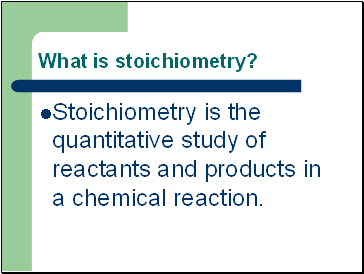## What is stoichiometry?

Stoichiometry is the quantitative study of reactants and products in a chemical reaction.

Slide 3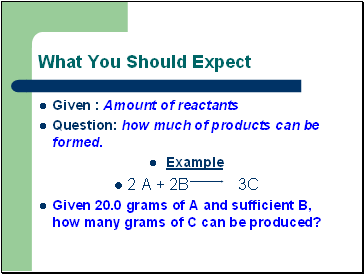What You Should Expect

Given : Amount of reactants

Question: how much of products can be formed.

Example

2 A + 2B 3C

Given 20.0 grams of A and sufficient B, how many grams of C can be produced?

Slide 4What do you need?

You will need to use

molar ratios,

molar masses,

balancing and interpreting equations, and

conversions between grams and moles.

Note: This type of problem is often called "mass-mass."

Slide 5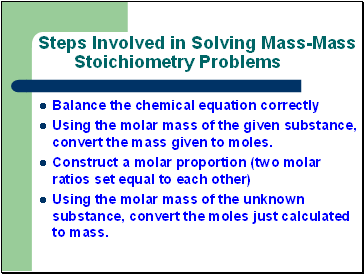## Steps Involved in Solving Mass-Mass Stoichiometry Problems

Balance the chemical equation correctly

Using the molar mass of the given substance, convert the mass given to moles.

Construct a molar proportion (two molar ratios set equal to each other)

Using the molar mass of the unknown substance, convert the moles just calculated to mass.

Slide 6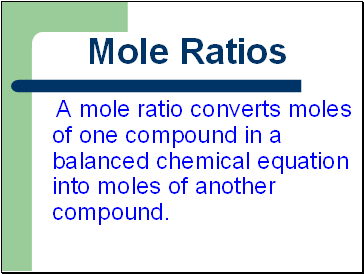## Mole Ratios

A mole ratio converts moles of one compound in a balanced chemical equation into moles of another compound.

Slide 7Example

Reaction between magnesium and oxygen to form magnesium oxide. ( fireworks)

2 Mg(s) + O2(g) 2 MgO(s)

Mole Ratios:

2 : 1 : 2

Slide 8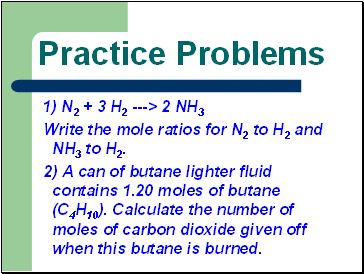## Practice Problems

1) N2 + 3 H2 ---> 2 NH3

Write the mole ratios for N2 to H2 and NH3 to H2.

2) A can of butane lighter fluid contains 1.20 moles of butane (C4H10). Calculate the number of moles of carbon dioxide given off when this butane is burned.

Slide 9## Mole-Mole Problems

Using the practice question 2) above:

Equation of reaction

2C4H10 + 13O2 8CO2 + 10H2O

Mole ratio

C4H10 CO2

1 : 4 [ bases]

1.2 : X [ problem]

By cross-multiplication, X = 4.8 mols of CO2 given off

Slide 10## Mole-Mass Problems

Problem 1: 1.50 mol of KClO3 decomposes. How many grams of O2 will be produced? [k = 39, Cl = 35.5, O = 16]

2 KClO3 2 KCl + 3 O2

Slide 11Go to page:
1  2  3